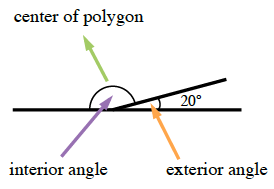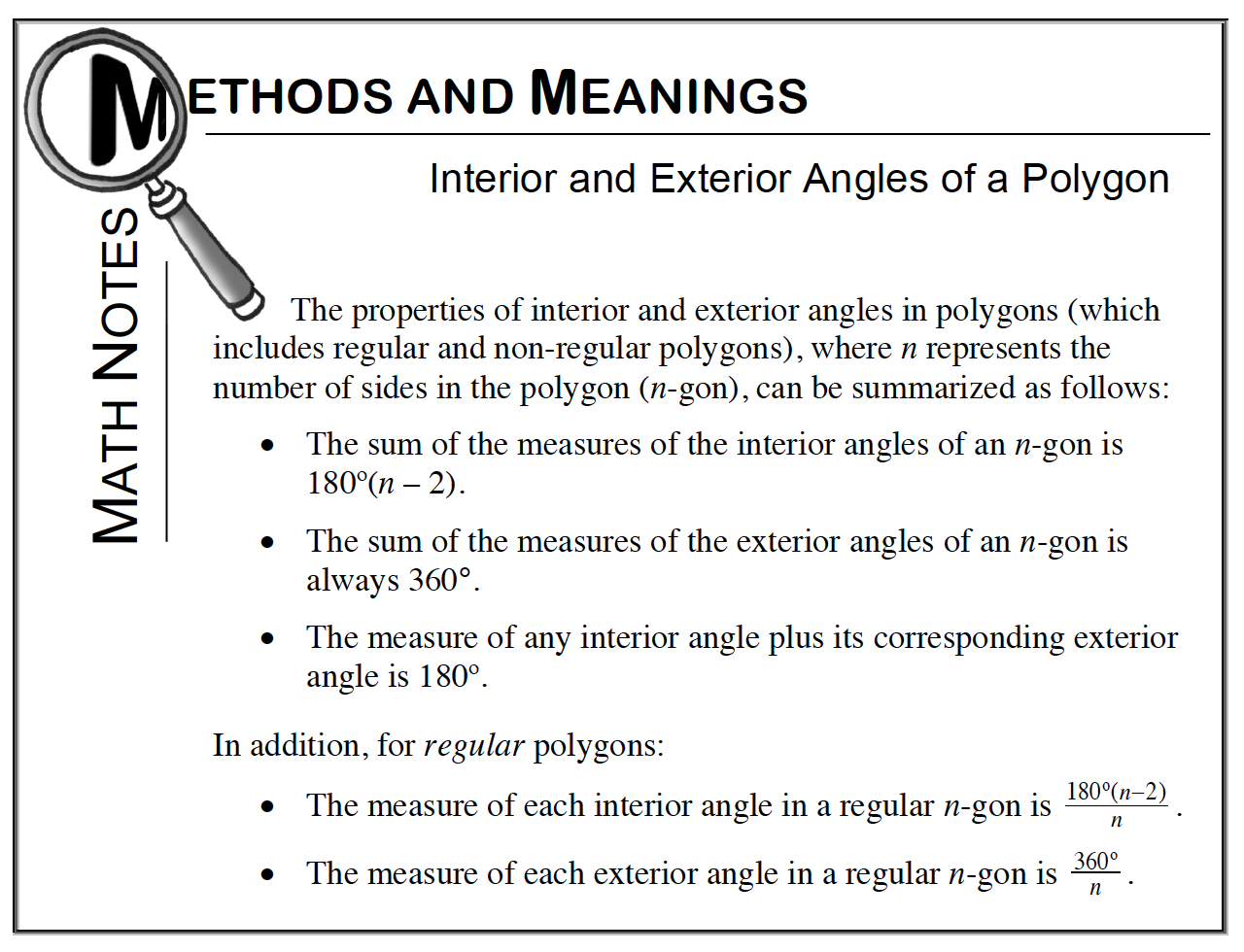### Home > INT2 > Chapter 8 > Lesson 8.3.1 > Problem8-58

8-58.

The exterior angle of a regular polygon is $20°$. Homework Help ✎

1. What is the measure of an interior angle of this polygon? Show how you know.

The interior and exterior angles are supplementary.2. How many sides does this polygon have? Show all work.

Refer to the Math Notes box below.The sum of the measures of the exterior angles is $360°$.

$18$